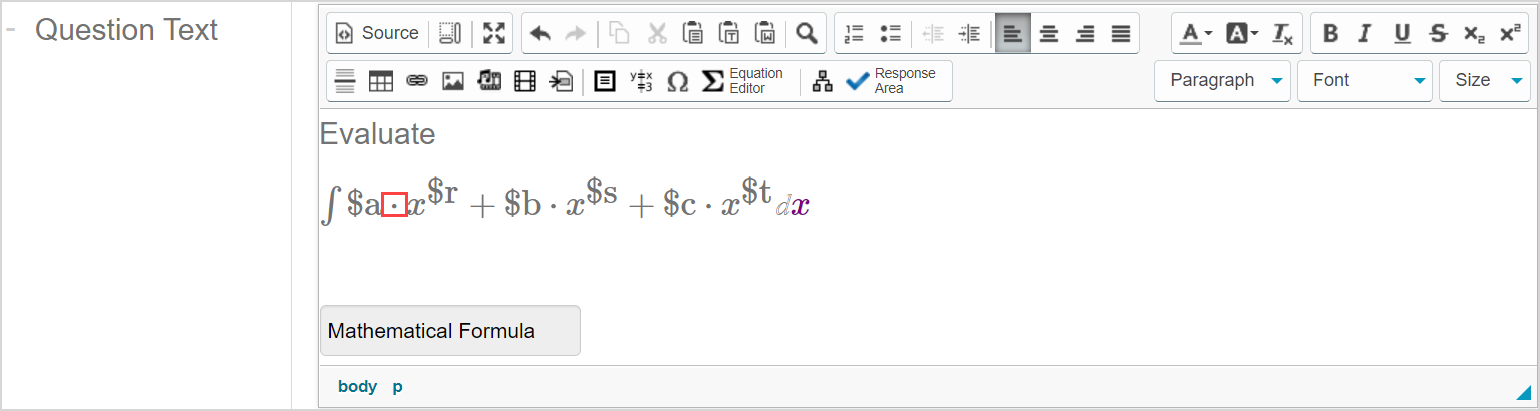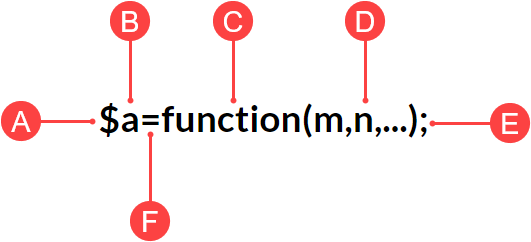# Follow Algorithm Editor syntax rules

Variables and functions that are entered into the Algorithm Editor to generate algorithmic questions must follow specific syntax rules to be properly interpreted by Möbius.

TIP: Alternatively, you can use the Algorithm Designer to create algorithms without needing to directly use Algorithm Editor syntax. Check out Create question algorithms using the Algorithm Designer.

## Syntax rules for variables

### Naming

Variables must first be defined for the function to be able to display randomly-generated values in the algorithmic question.

The naming of your variable must following these rules:

• Must be of the form \$name.
• String name can consist of letters (a-z and A-Z), numbers (0-9), and the underscore character (_).
• First character of the name must be an alphabetic character (a-z or A-Z).
• The variable e should not be assigned as a global variable in Maple code (it can be used, however, as a local variable).

### Structure

The use of the dollar sign character (\$) is reserved for introducing an algorithmic variable.

TIP: Use \\$ to insert the actual dollar sign character. The use of the backslash character (\) is reserved as an escape character in algorithmic command syntax. Use \\ to insert the actual backslash character.

Variables can be:

• Fixed strings (Example\$a="triangle";)
• Fixed numbers (Example\$a=5;)
• Other variables (Example\$a=\$m;)
• Mathematical expressions (Example\$a=x^2-3*x+4;)
• Functions (Example\$a=sig(3, 20.8571);)

TIP: Be sure to separate algorithmic variable names from other variables in your question statement with the multiplication symbol (•) when using algorithmic variables in the Equation Editor. This symbol can be inserted by pressing Shift+* on your keyboard.Otherwise, the variable from your question statement will be interpreted as a part of the algorithmic variable.

### Usage

You can define as many variables as needed to author your perfect algorithmic question.

The defined algorithmic variable can then be inserted into question text.

TIP: You can also combine the variable with available functions to transform a static question into an algorithmic question (check out Author an algorithmic question).

You can enter your defined variable(s) in other areas associated with question authoring beyond the question text such as:

• Feedback
• Hints

TIP: You can define an algorithmic variable inline to simplify an algorithmic variable expression and use it outside of the Algorithm Editor. The syntax to enclose the expression is \${ … }. Example — You could define a variable in the Algorithm Editor as \$n=5;. Entering \${\$n + 1} in the Question Text field will then display 6 as the question text.

NOTE: Variables can't be redefined in terms of themselves. Example — The statement \$a=\$a+1; is invalid.

## Syntax rules for functions

### Naming

Check out View available functions for algorithmic questions for a comprehensive list of the available functions that can be used for generating algorithmic questions.

### Structure

The general structure for an algorithmic command with a function in the Algorithm Editor is:A.Dollar sign \$ Character used to introduce the algorithmic variable in the command line.

B.Variable name — Character(s) used to name the algorithmic variable.

C.Function — Algorithmic function name.

D.Placeholder — Placeholder(s) that represent the number(s) or string that you want to use for your algorithmic question that are acted upon by C.

E.Semicolon ; Character to be used at the end of every algorithmic command.

F. Equal sign = Character to separate the algorithmic variable from the function.

### Usage

You can enter as many command lines (consisting of variables and functions) as needed to author your perfect algorithmic question.